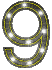/NINE - NINE     According to numerologists,   the number nine holds special rank.     It is associated with forgiveness,   compassion   and success on the positive side   and arrogance   and self-righteousness on the negative side.     9 is an important number in many spiritual communities,   and hence the Gregorian date of September 9th.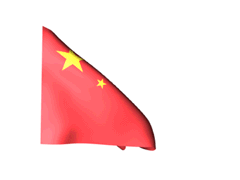The Chinese associated the number nine with long life,   the pronunciation of word for 9 is similar to the word for long-lasting.     Ancient Chinese emperors associated themselves closely with the number nine,   which historically appeared in architecture   and royal dress,   often in the form of nine fearsome dragons.     The dragon often symbolizes the Emperor,   and the number nine can be found in many ornaments in the Beijing's Forbidden City.     The palace complex at the Forbidden City is said to have been built with 9,999 rooms.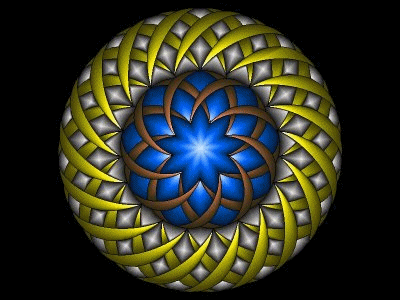In the Bahá'í Faith,   9 symbolizes completeness.     In addition,   the word Bahá' in the Abjad notation has a value of 9,   and a 9-pointed star is used to symbolize the religion.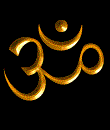In Hinduism,   The number 9 is revered and is considered a complete,   perfected and divine number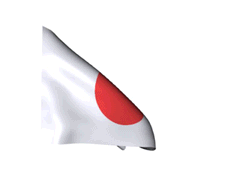In Japanese,   the word for nine is a homophone for the word for suffering,   so the number is considered highly unlucky,   second to four,   which sounds like death.     Many Japanese will avoid room numbers or floors including nine at hotels or hospitals,   if they havent allready been eliminated.           Pythagoras,   the Greek mathematician and origin of the famous Pythagorean theorem,   noted that nine has many unique properties.     If you multiply nine by any two,   three   or four-digit number,   the sums of those can always broken down to nine such as   9 × 9 = 81   (8 + 1 = 9)       9 x 62 = 558   (5+5+8=18,   1+8=9)       9 X 234 = 2106   (2 + 1 + 0 + 6 = 9)         Also,   Two positive integers that are transpositions of each other   create a number that is a whole multiple of nine.     52 - 25 = 27   (2 + 7 = 9)       The numbers can be transposed to any position....     4436273 - 2367434 = 2068839   (2+0+6+8+8+3+9=36,   3+6=9)       This works no matter how many digits that are transposed...     4436273 - 4432673=3600   (3+6+0+0=9)         9 has other sinteresting properties...     12345679 x 9 = 111111111     12345679 x 18 = 222222222     12345679 x 81 = 999999999         Six consecutive nines appear in the decimal places 762 through 767 of pi.     This is known as the Feynman point.